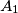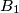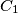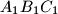### IMO Shortlist 2013 problem G6

Kvaliteta:
Avg: 4,0
Težina:
Avg: 8,0
Let the excircle of the triangle$ABC$ lying opposite to$A$ touch its side$BC$ at the point$A_1$. Define the points$B_1$ and$C_1$ analogously. Suppose that the circumcentre of the triangle$A_1B_1C_1$ lies on the circumcircle of the triangle$ABC$. Prove that the triangle$ABC$ is right-angled.
Izvor: Russia